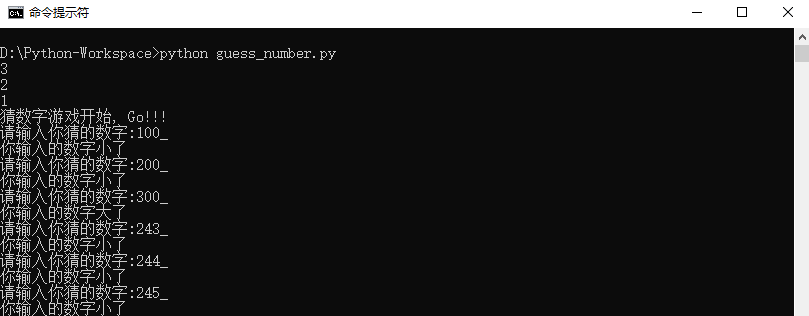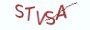Python项目实战

# 简单的猜数字游戏

```# 导入random模块
import random
# 获取从1到100000之间的随机数
random_number = random.randint(1, 1000000)
# 执行input方法获取用户的输入，input的返回值为字符串类型，通过int()将其转换为整型
guess_number = int(input("Please enter the number:____\b\b\b\b"))```

# 程序源码

```# __author__ = 薯条老师
# __referrer__ = http://chipscoco.com/?cate=59
import time
import random

def get_random_number(start=0, end=10**3):
return random.randint(start, end)

def countdown(seconds=3, message=""):
"""
:param seconds: 倒数的秒数
:param message:  倒计时结束后输出的提示信息
:return:
"""
for _ in range(seconds, 0, -1):
_, _ = print(_), time.sleep(1)
else:
print(message)

def serve_forever():

random_number = get_random_number()
countdown(message="猜数字游戏开始，Go!!!")

while True:
try:
guess_number = int(input("请输入你猜的数字:"+"_"*4+"\b"*4))
except ValueError:
print("请输入合法的数字！")
continue
if guess_number != random_number:
print("你输入的数字大了") if guess_number > random_number else print("你输入的数字小了")
continue
print("恭喜你猜对了！！！")
if input("按键盘任意键继续玩猜数字游戏或输入quit退出游戏:____\b\b\b\b").lower() == "quit":
break
else:
random_number = get_random_number()
print("游戏已被终止，再见！！！")

if __name__ == "__main__":
serve_forever()```# 最具实力的小班培训

`(1) Python后端工程师高薪就业班，月薪11K-18K，免费领取课程大纲(2) Python爬虫工程师高薪就业班，年薪十五万，免费领取课程大纲(3) Java后端开发工程师高薪就业班，月薪11K-20K, 免费领取课程大纲(4) Python大数据分析，量化投资就业班，月薪12K-25K,免费领取课程大纲`## 注册账号